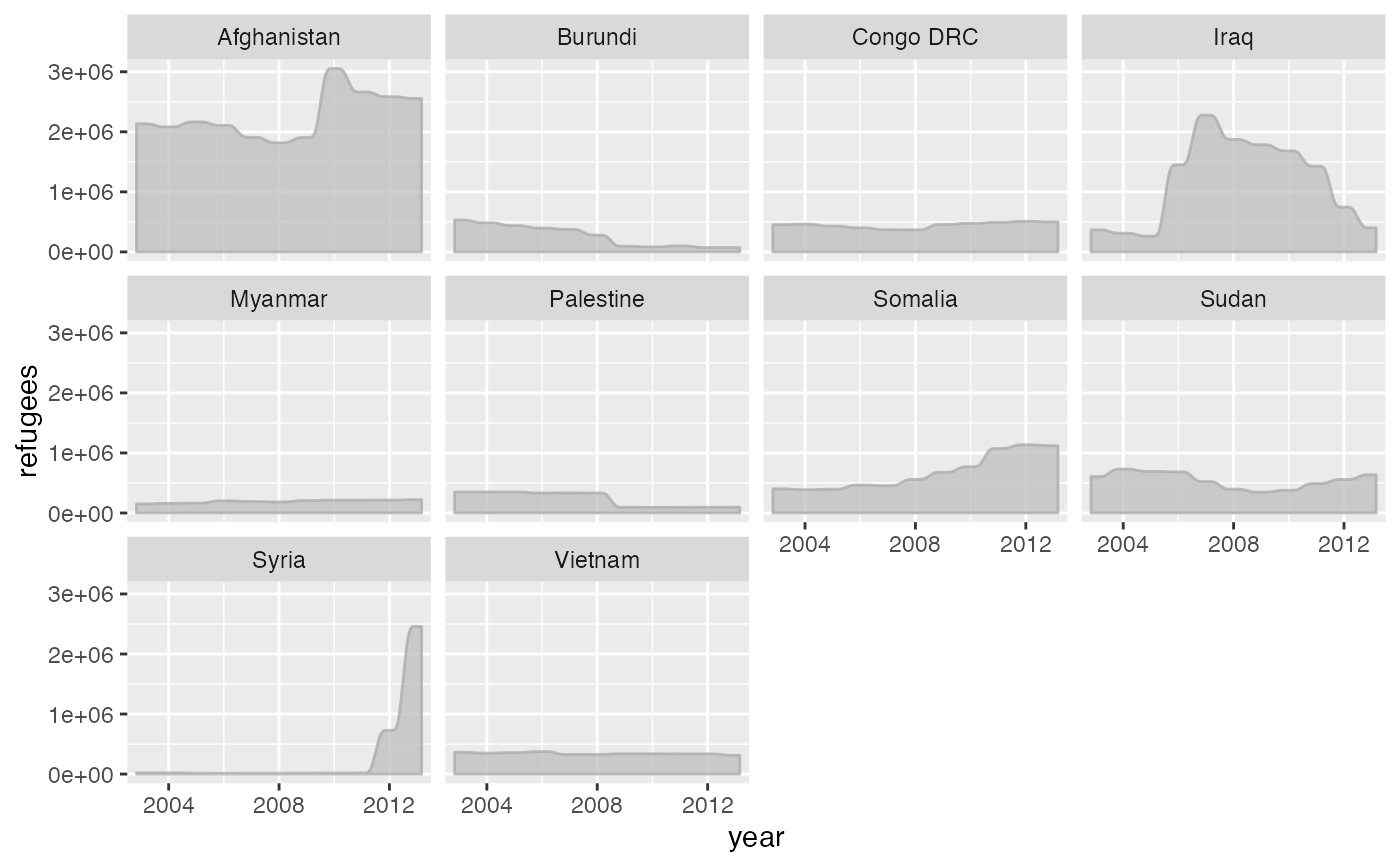Given a dataset with alluvial structure, stat_alluvium calculates the centroids (x and y) and heights (ymin and ymax) of the lodes, the intersections of the alluvia with the strata. It leverages the group aesthetic for plotting purposes (for now).

stat_alluvium(
mapping = NULL,
data = NULL,
geom = "alluvium",
position = "identity",
decreasing = NULL,
reverse = NULL,
absolute = NULL,
discern = FALSE,
negate.strata = NULL,
aggregate.y = NULL,
cement.alluvia = NULL,
lode.guidance = NULL,
lode.ordering = NULL,
aes.bind = NULL,
infer.label = FALSE,
min.y = NULL,
max.y = NULL,
na.rm = FALSE,
show.legend = NA,
inherit.aes = TRUE,
...
)

## Arguments

mapping

Set of aesthetic mappings created by aes(). If specified and inherit.aes = TRUE (the default), it is combined with the default mapping at the top level of the plot. You must supply mapping if there is no plot mapping.

data

The data to be displayed in this layer. There are three options:

If NULL, the default, the data is inherited from the plot data as specified in the call to ggplot().

A data.frame, or other object, will override the plot data. All objects will be fortified to produce a data frame. See fortify() for which variables will be created.

A function will be called with a single argument, the plot data. The return value must be a data.frame, and will be used as the layer data. A function can be created from a formula (e.g. ~ head(.x, 10)).

geom

The geometric object to use display the data; override the default.

position

Position adjustment, either as a string naming the adjustment (e.g. "jitter" to use position_jitter), or the result of a call to a position adjustment function. Use the latter if you need to change the settings of the adjustment.

decreasing

Logical; whether to arrange the strata at each axis in the order of the variable values (NA, the default), in ascending order of totals (largest on top, FALSE), or in descending order of totals (largest on bottom, TRUE).

reverse

Logical; if decreasing is NA, whether to arrange the strata at each axis in the reverse order of the variable values, so that they match the order of the values in the legend. Ignored if decreasing is not NA. Defaults to TRUE.

absolute

Logical; if some cases or strata are negative, whether to arrange them (respecting decreasing and reverse) using negative or absolute values of y.

discern

Passed to to_lodes_form() if data is in alluvia format.

negate.strata

A vector of values of the stratum aesthetic to be treated as negative (will ignore missing values with a warning).

aggregate.y

Deprecated alias for cement.alluvia.

cement.alluvia

Logical value indicating whether to aggregate y values over equivalent alluvia before computing lode and flow positions.

lode.guidance

The function to prioritize the axis variables for ordering the lodes within each stratum, or else a character string identifying the function. Character options are "zigzag", "frontback", "backfront", "forward", and "backward" (see lode-guidance-functions).

lode.ordering

Deprecated in favor of the order aesthetic. A list (of length the number of axes) of integer vectors (each of length the number of rows of data) or NULL entries (indicating no imposed ordering), or else a numeric matrix of corresponding dimensions, giving the preferred ordering of alluvia at each axis. This will be used to order the lodes within each stratum by sorting the lodes first by stratum, then by the provided vectors, and lastly by remaining factors (if the vectors contain duplicate entries and therefore do not completely determine the lode orderings).

aes.bind

At what grouping level, if any, to prioritize differentiation aesthetics when ordering the lodes within each stratum. Defaults to "none" (no aesthetic binding) with intermediate option "flows" to bind aesthetics after stratifying by axes linked to the index axis (the one adjacent axis in stat_flow(); all remaining axes in stat_alluvium()) and strongest option "alluvia" to bind aesthetics after stratifying by the index axis but before stratifying by linked axes (only available for stat_alluvium()). Stratification by any axis is done with respect to the strata at that axis, after separating positive and negative strata, consistent with the values of decreasing, reverse, and absolute. Thus, if "none", then lode orderings will not depend on aesthetic variables. All aesthetic variables are used, in the order in which they are specified in aes().

infer.label

Logical; whether to assign the stratum or alluvium variable to the label aesthetic. Defaults to FALSE, and requires that no label aesthetic is assigned. This parameter is intended for use only with data in alluva form, which are converted to lode form before the statistical transformation. Deprecated; use ggplot2::after_stat() instead.

min.y, max.y

Numeric; bounds on the heights of the strata to be rendered. Use these bounds to exclude strata outside a certain range, for example when labeling strata using ggplot2::geom_text().

na.rm

Logical: if FALSE, the default, NA lodes are not included; if TRUE, NA lodes constitute a separate category, plotted in grey (regardless of the color scheme).

show.legend

logical. Should this layer be included in the legends? NA, the default, includes if any aesthetics are mapped. FALSE never includes, and TRUE always includes. It can also be a named logical vector to finely select the aesthetics to display.

inherit.aes

If FALSE, overrides the default aesthetics, rather than combining with them. This is most useful for helper functions that define both data and aesthetics and shouldn't inherit behaviour from the default plot specification, e.g. borders().

...

Additional arguments passed to ggplot2::layer().

## Aesthetics

stat_alluvium, stat_flow, and stat_stratum require one of two sets of aesthetics:

• x and at least one of alluvium and stratum

• any number of axis[0-9]* (axis1, axis2, etc.)

Use x, alluvium, and/or stratum for data in lodes format and axis[0-9]* for data in alluvia format (see alluvial-data). Arguments to parameters inconsistent with the format will be ignored. Additionally, each stat_*() accepts the following optional aesthetics:

• y

• weight

• order

• group

• label

y controls the heights of the alluvia, and may be aggregated across equivalent observations. weight applies to the computed variables (see that section below) but does not affect the positional aesthetics. order, recognized by stat_alluvium() and stat_flow(), is used to arrange the lodes within each stratum. It tolerates duplicates and takes precedence over the differentiation aesthetics (when aes.bind is not "none") and lode guidance with respect to the remaining axes. (It replaces the deprecated parameter lode.ordering.) group is used internally; arguments are ignored. label is used to label the strata or lodes and must take a unique value across the observations within each stratum or lode.

These and any other aesthetics are aggregated as follows: Numeric aesthetics, including y, are summed. Character and factor aesthetics, including label, are assigned to strata or lodes provided they take unique values across the observations within each (and are otherwise assigned NA).

## Computed variables

These can be used with ggplot2::after_stat() to control aesthetic evaluation.

n

number of cases in lode

count

cumulative weight of lode

prop

weighted proportion of lode

stratum

value of variable used to define strata

deposit

order in which (signed) strata are deposited

lode

lode label distilled from alluvia (stat_alluvium() and stat_flow() only)

flow

direction of flow "to" or "from" from its axis (stat_flow() only)

The numerical variables n, count, and prop are calculated after the data are grouped by x and weighted by weight (in addition to y). The integer variable deposit is used internally to sort the data before calculating heights. The character variable lode is obtained from alluvium according to distill.

## Package options

stat_stratum, stat_alluvium, and stat_flow order strata and lodes according to the values of several parameters, which must be held fixed across every layer in an alluvial plot. These package-specific options set global values for these parameters that will be defaulted to when not manually set:

• ggalluvial.decreasing (each stat_*): defaults to NA.

• ggalluvial.reverse (each stat_*): defaults to TRUE.

• ggalluvial.absolute (each stat_*): defaults to TRUE.

• ggalluvial.cement.alluvia (stat_alluvium): defaults to FALSE.

• ggalluvial.lode.guidance (stat_alluvium): defaults to "zigzag".

• ggalluvial.aes.bind (stat_alluvium and stat_flow): defaults to "none".

See base::options() for how to use options.

## Defunct parameters

The previously defunct parameters weight and aggregate.wts have been discontinued. Use y and cement.alluvia instead.

ggplot2::layer() for additional arguments and geom_alluvium(), geom_lode(), and geom_flow() for the corresponding geoms.

Other alluvial stat layers: stat_flow(), stat_stratum()

## Examples

# illustrate positioning
ggplot(as.data.frame(Titanic),
aes(y = Freq,
axis1 = Class, axis2 = Sex, axis3 = Age,
color = Survived)) +
stat_stratum(geom = "errorbar") +
geom_line(stat = "alluvium") +
stat_alluvium(geom = "pointrange") +
geom_text(stat = "stratum", aes(label = after_stat(stratum))) +
scale_x_discrete(limits = c("Class", "Sex", "Age"))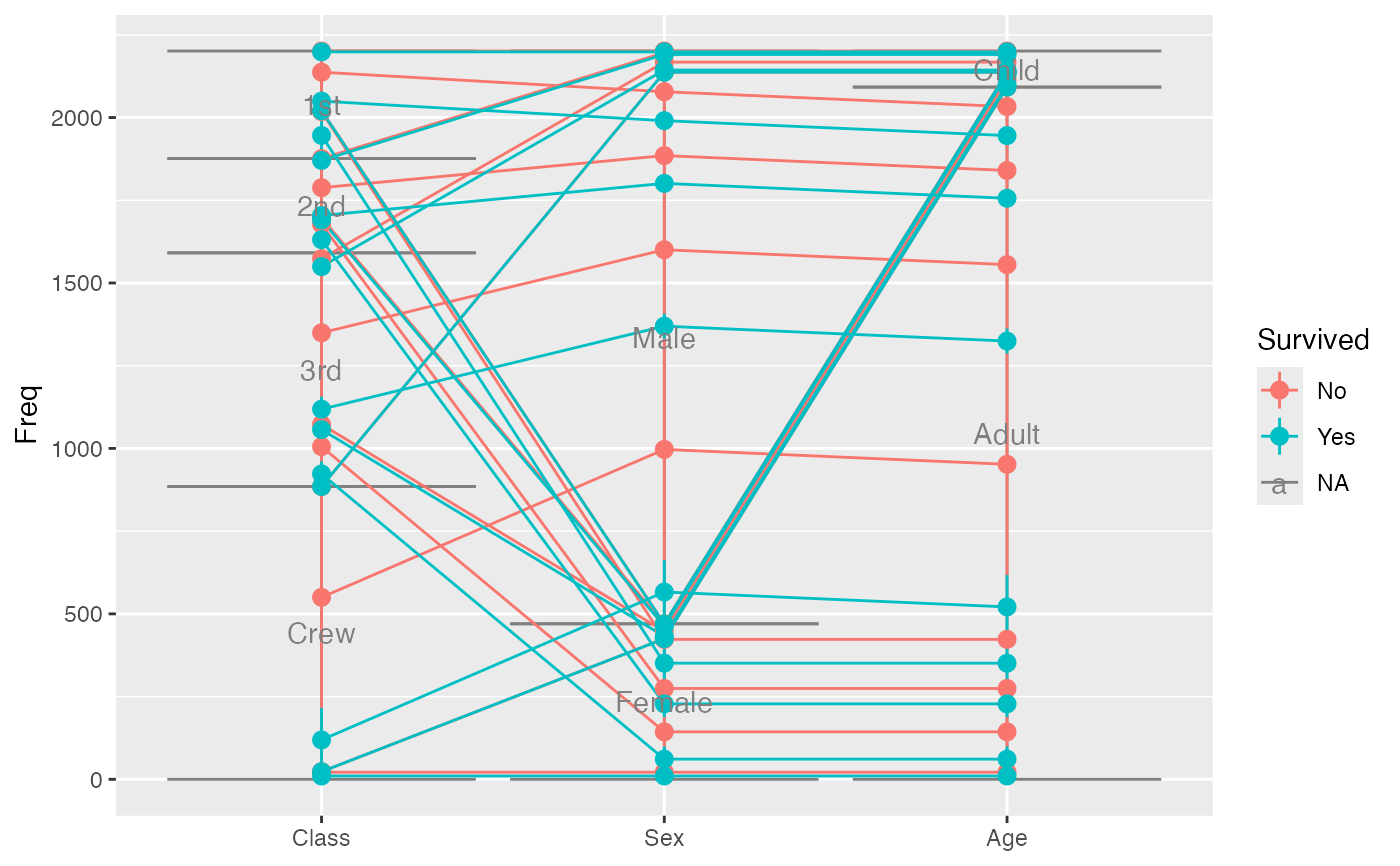# lode ordering examples
gg <- ggplot(as.data.frame(Titanic),
aes(y = Freq,
axis1 = Class, axis2 = Sex, axis3 = Age)) +
geom_stratum() +
geom_text(stat = "stratum", aes(label = after_stat(stratum))) +
scale_x_discrete(limits = c("Class", "Sex", "Age"))
# use of lode controls
gg + geom_flow(aes(fill = Survived, alpha = Sex), stat = "alluvium",
lode.guidance = "forward")
#> Warning: Using alpha for a discrete variable is not advised.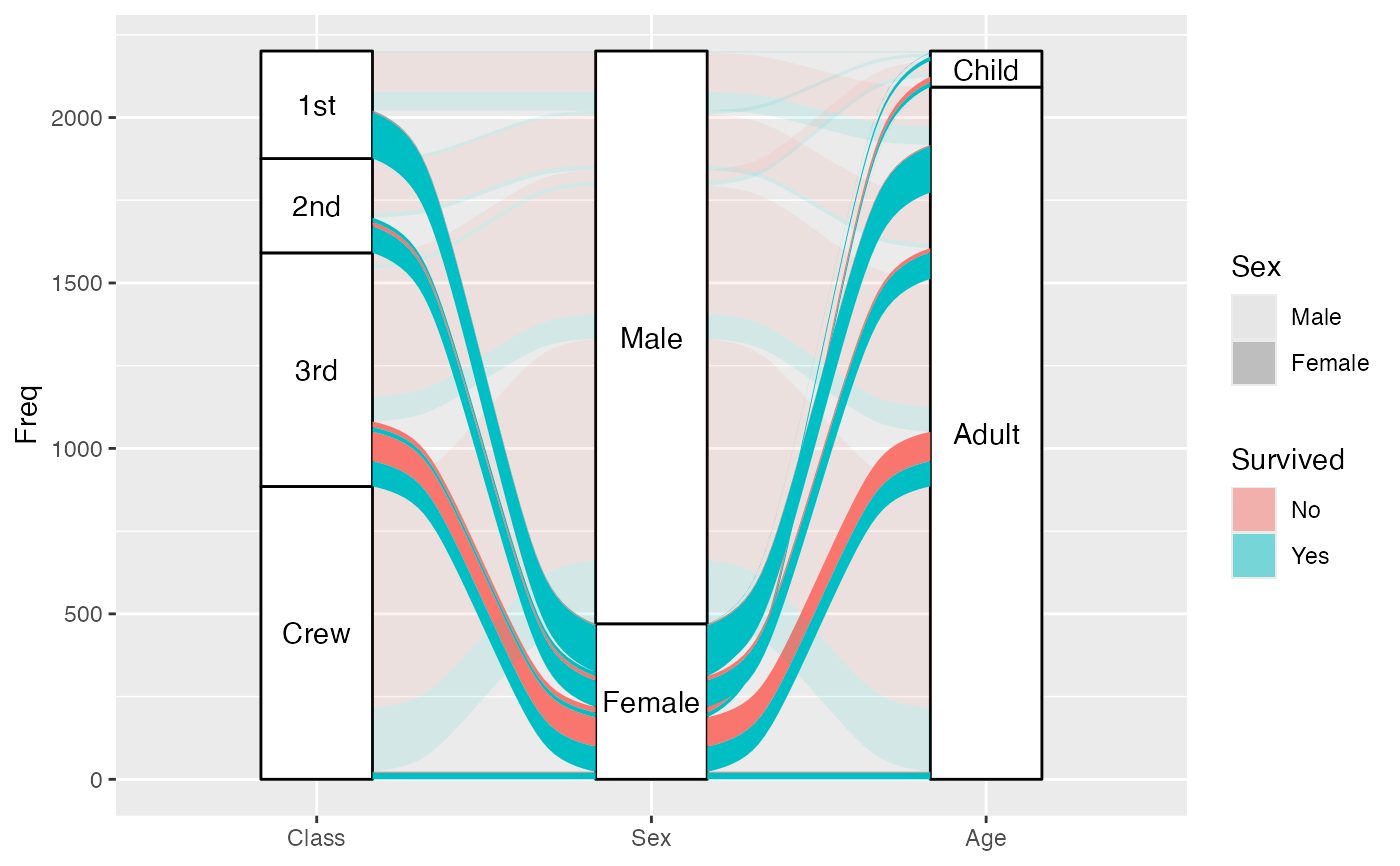# prioritize aesthetic binding
gg + geom_flow(aes(fill = Survived, alpha = Sex), stat = "alluvium",
aes.bind = "alluvia", lode.guidance = "forward")
#> Warning: Using alpha for a discrete variable is not advised.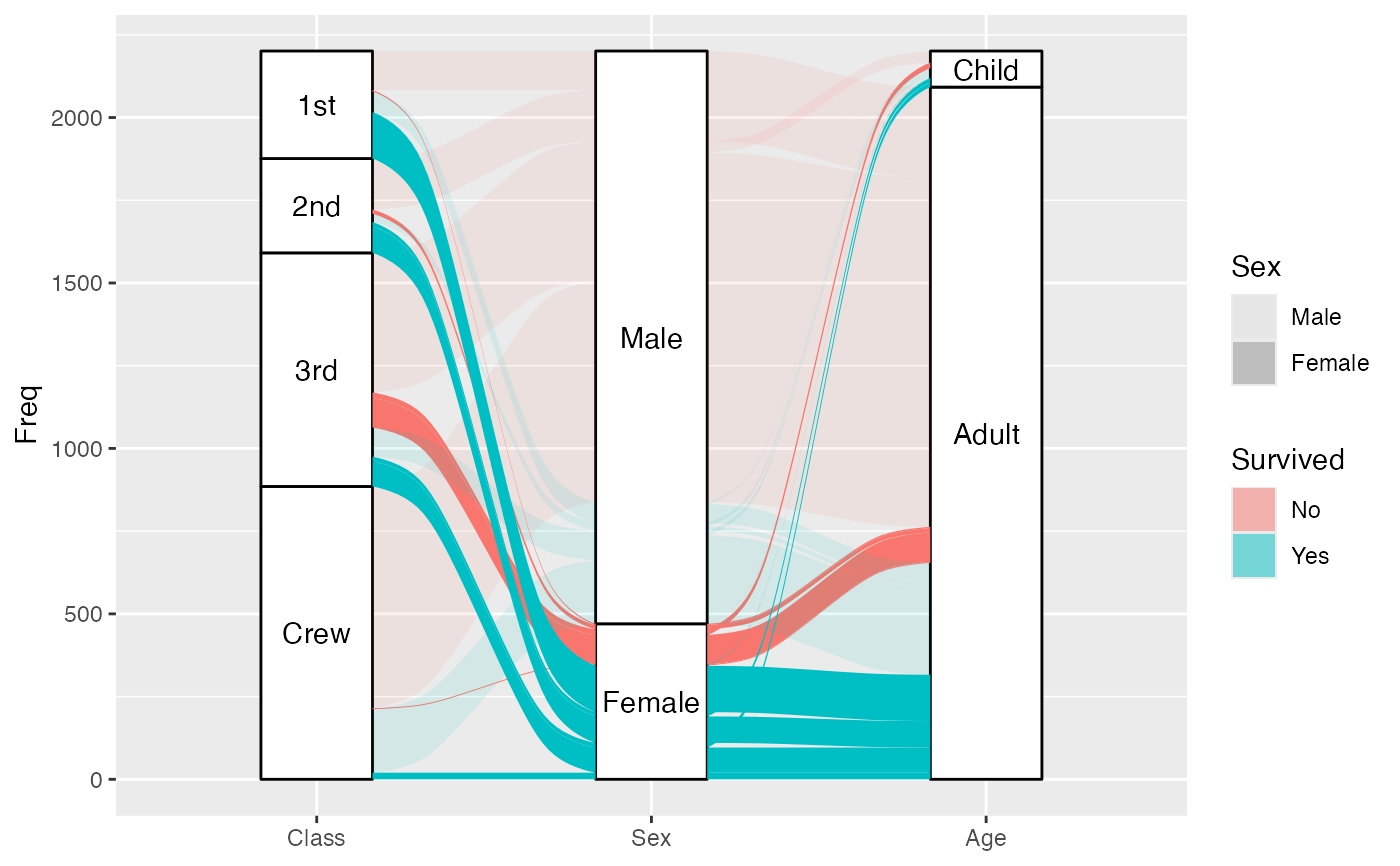# use of custom lode order
gg + geom_flow(aes(fill = Survived, alpha = Sex, order = sample(x = 32)),
stat = "alluvium")
#> Warning: Ignoring unknown aesthetics: order
#> Warning: Using alpha for a discrete variable is not advised.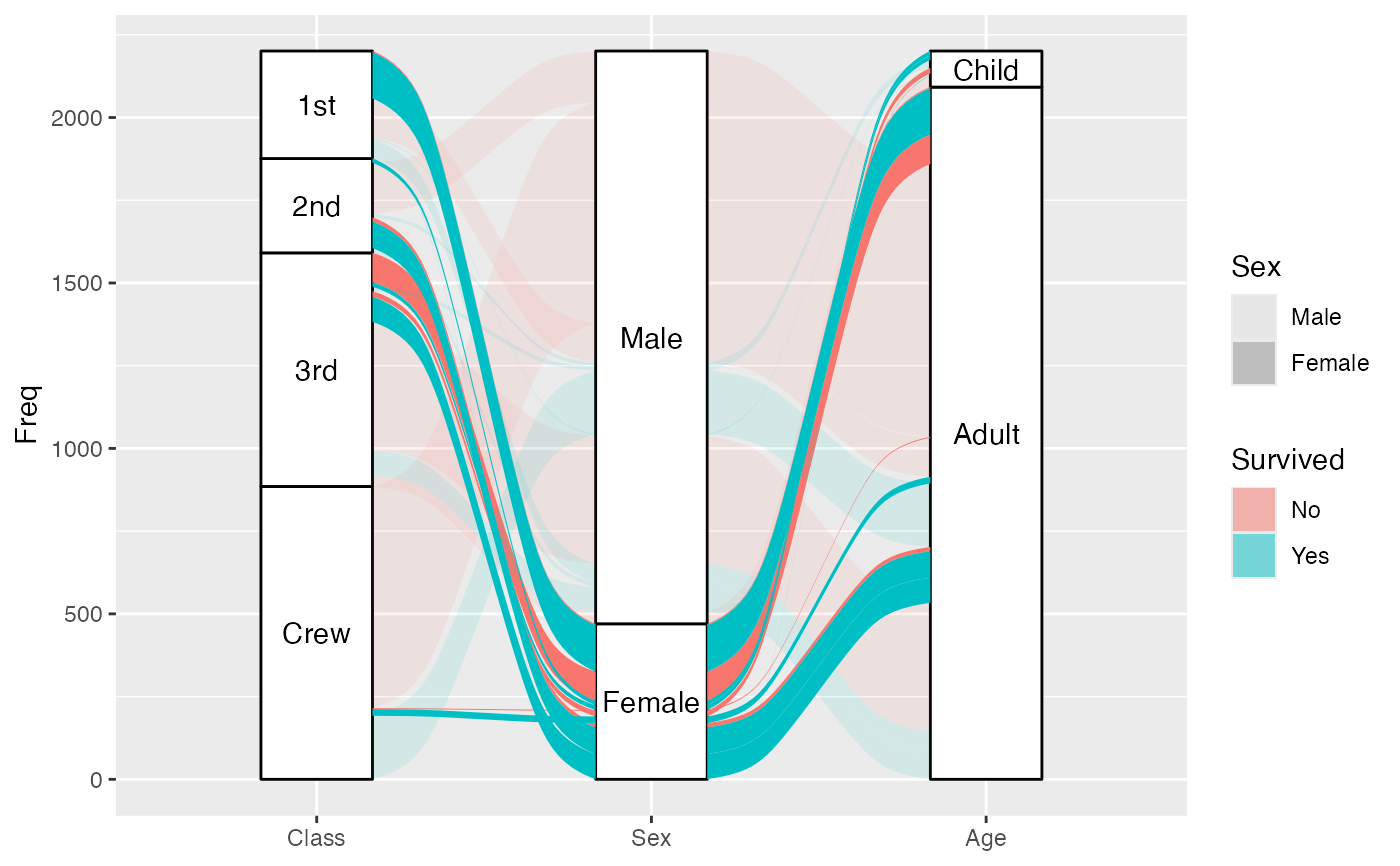# use of custom luide guidance function
lode_custom <- function(n, i) {
stopifnot(n == 3)
switch(
i,
1 = 1:3,
2 = c(2, 3, 1),
3 = 3:1
)
}
gg + geom_flow(aes(fill = Survived, alpha = Sex), stat = "alluvium",
aes.bind = "flow", lode.guidance = lode_custom)
#> Warning: Using alpha for a discrete variable is not advised.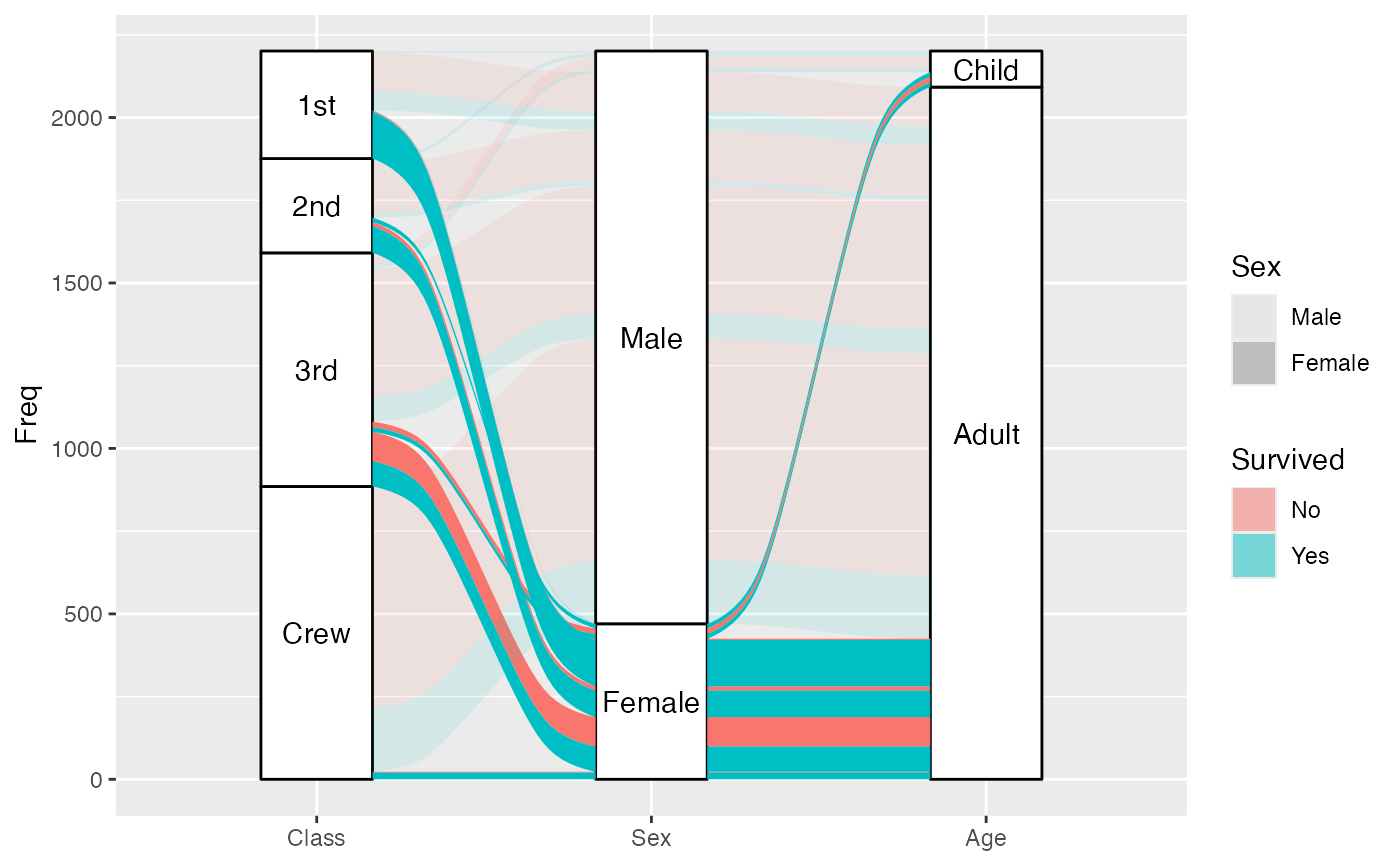# omit missing elements & reverse the y axis
ggplot(ggalluvial::majors,
aes(x = semester, stratum = curriculum, alluvium = student, y = 1)) +
geom_alluvium(fill = "darkgrey", na.rm = TRUE) +
geom_stratum(aes(fill = curriculum), color = NA, na.rm = TRUE) +
theme_bw() +
scale_y_reverse()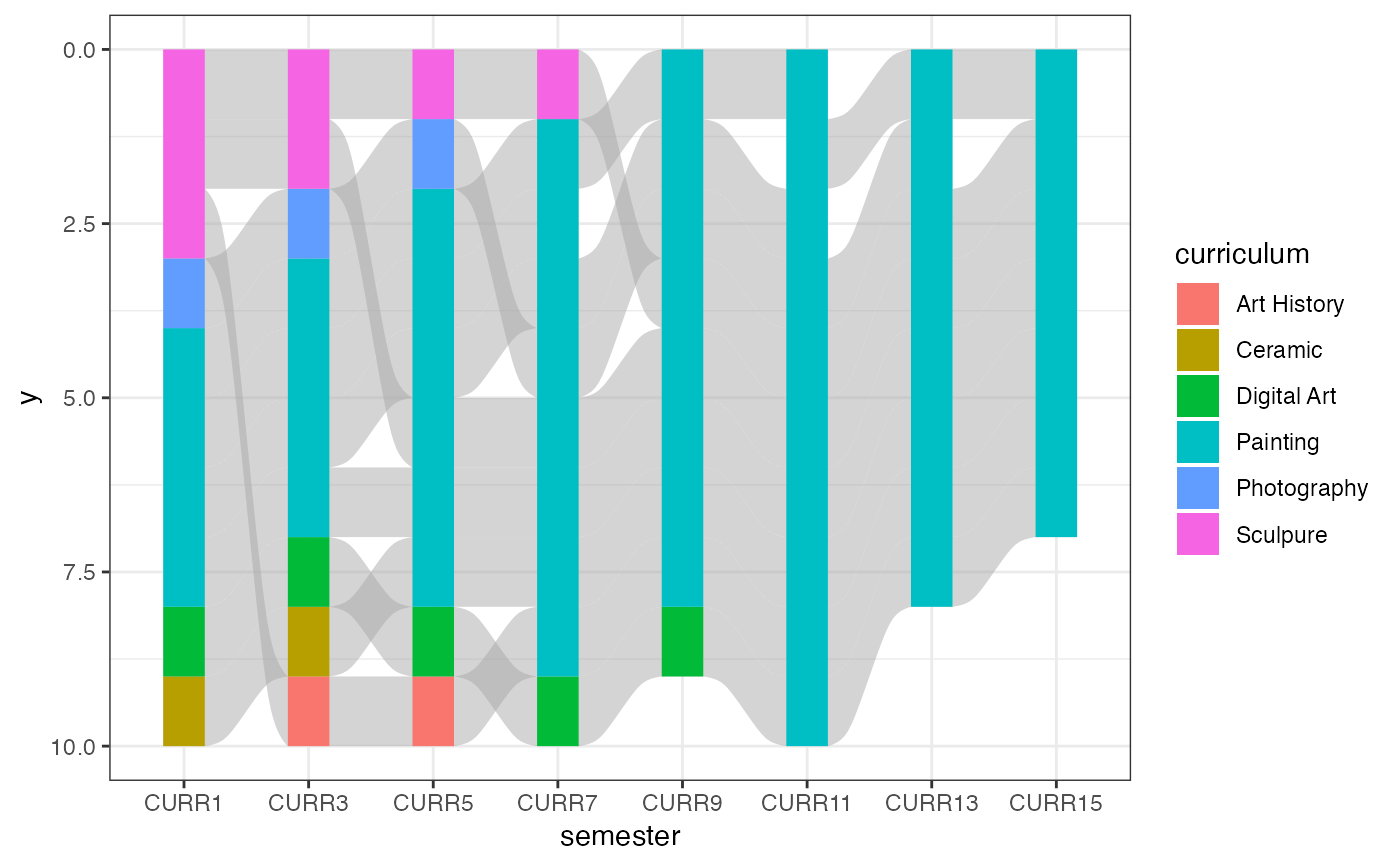# \donttest{
# alluvium cementation examples
gg <- ggplot(ggalluvial::majors,
aes(x = semester, stratum = curriculum, alluvium = student,
fill = curriculum)) +
geom_stratum()
# diagram with outlined alluvia and labels
gg + geom_flow(stat = "alluvium", color = "black") +
geom_text(aes(label = after_stat(lode)), stat = "alluvium")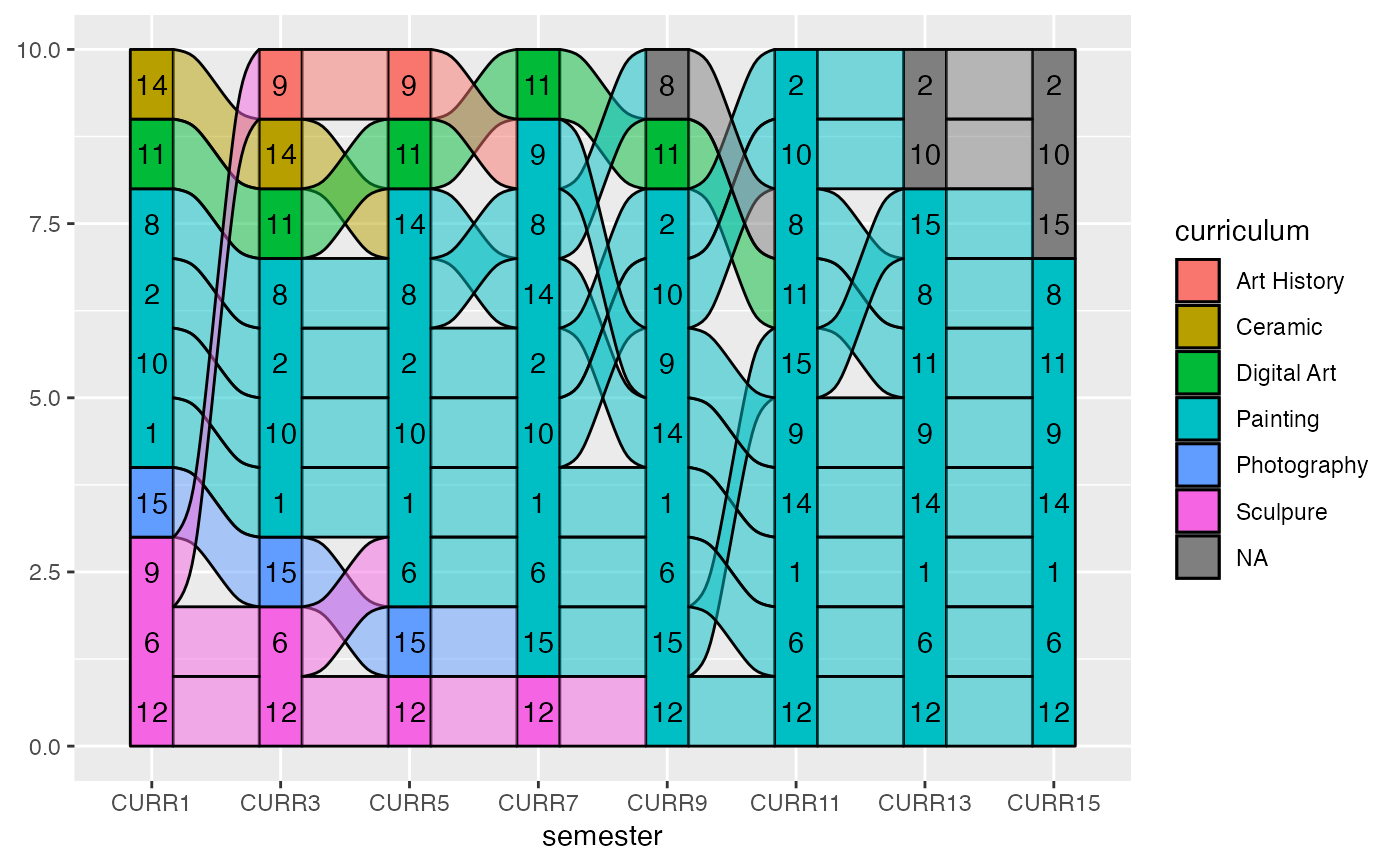# cemented diagram with default distillation (first most common alluvium)
gg +
geom_flow(stat = "alluvium", color = "black", cement.alluvia = TRUE) +
geom_text(aes(label = after_stat(lode)), stat = "alluvium",
cement.alluvia = TRUE)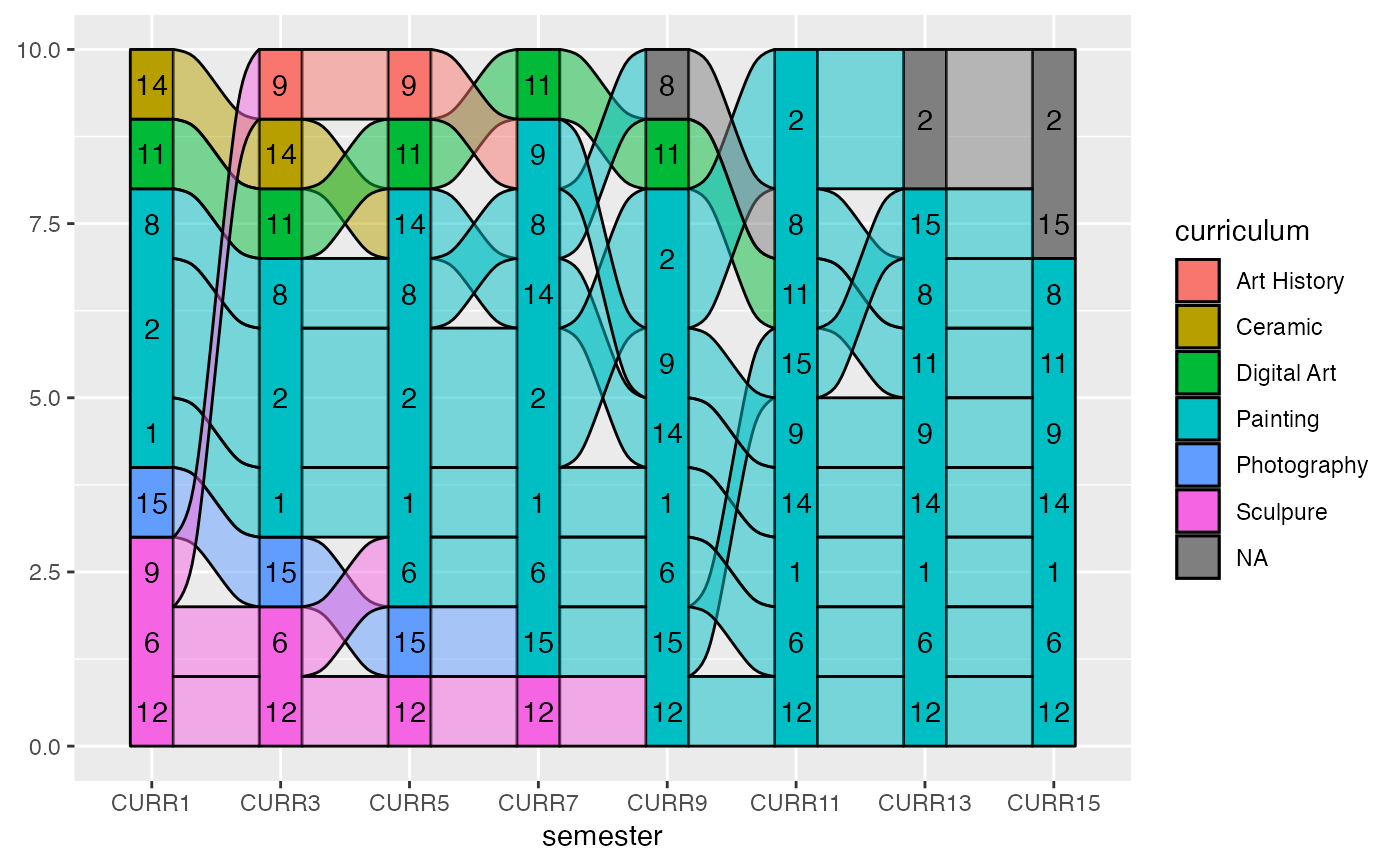# cemented diagram with custom label distillation
gg +
geom_flow(stat = "alluvium", color = "black", cement.alluvia = TRUE) +
geom_text(aes(label = after_stat(lode)), stat = "alluvium",
cement.alluvia = TRUE,
distill = function(x) paste(x, collapse = "; "))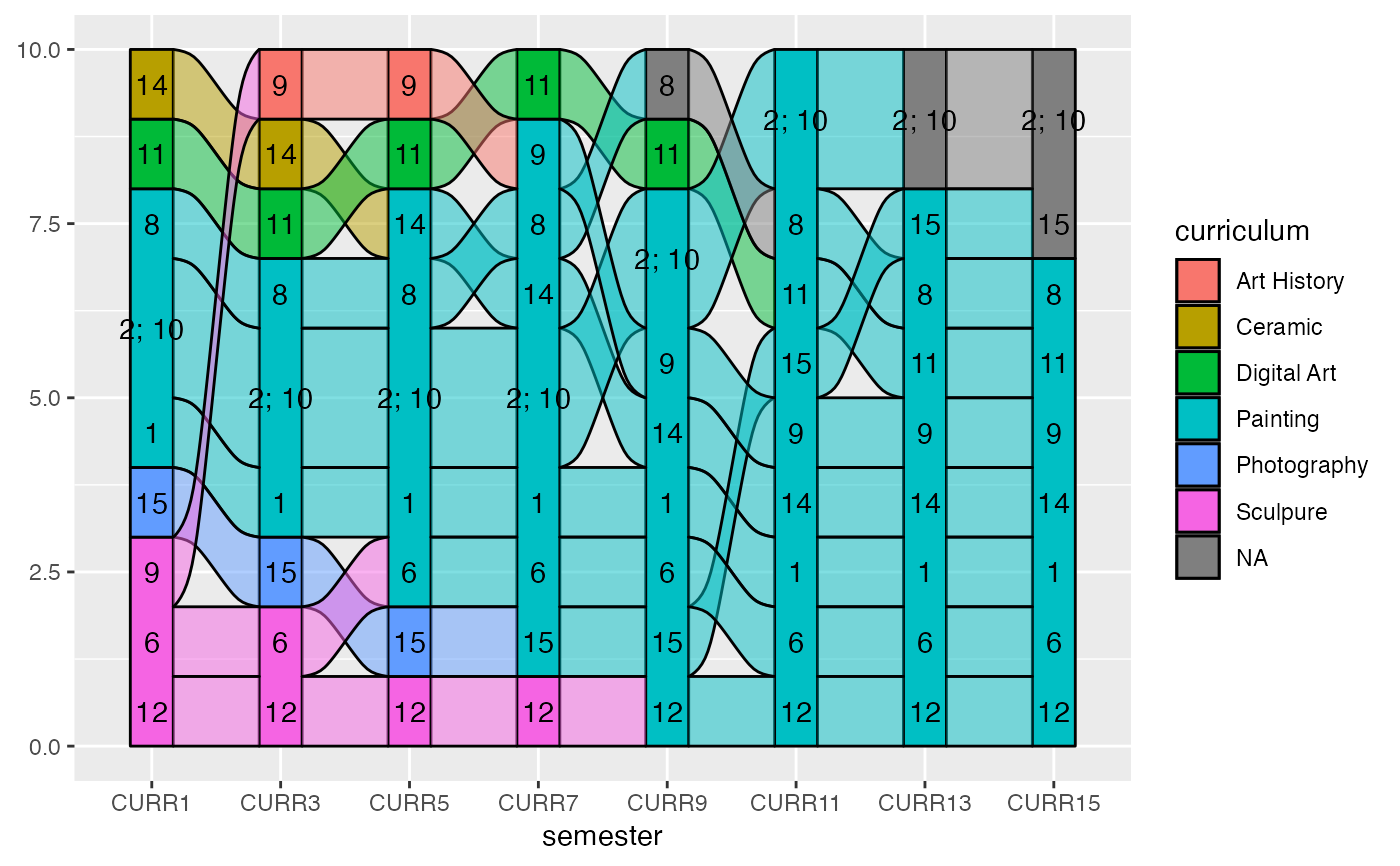# }

# \donttest{
data(babynames, package = "babynames")
# a discontiguous alluvium
bn <- subset(babynames, prop >= .01 & sex == "F" & year > 1962 & year < 1968)
ggplot(data = bn,
aes(x = year, alluvium = name, y = prop)) +
geom_alluvium(aes(fill = name, color = name == "Tammy"),
decreasing = TRUE, show.legend = FALSE) +
scale_color_manual(values = c("#00000000", "#000000"))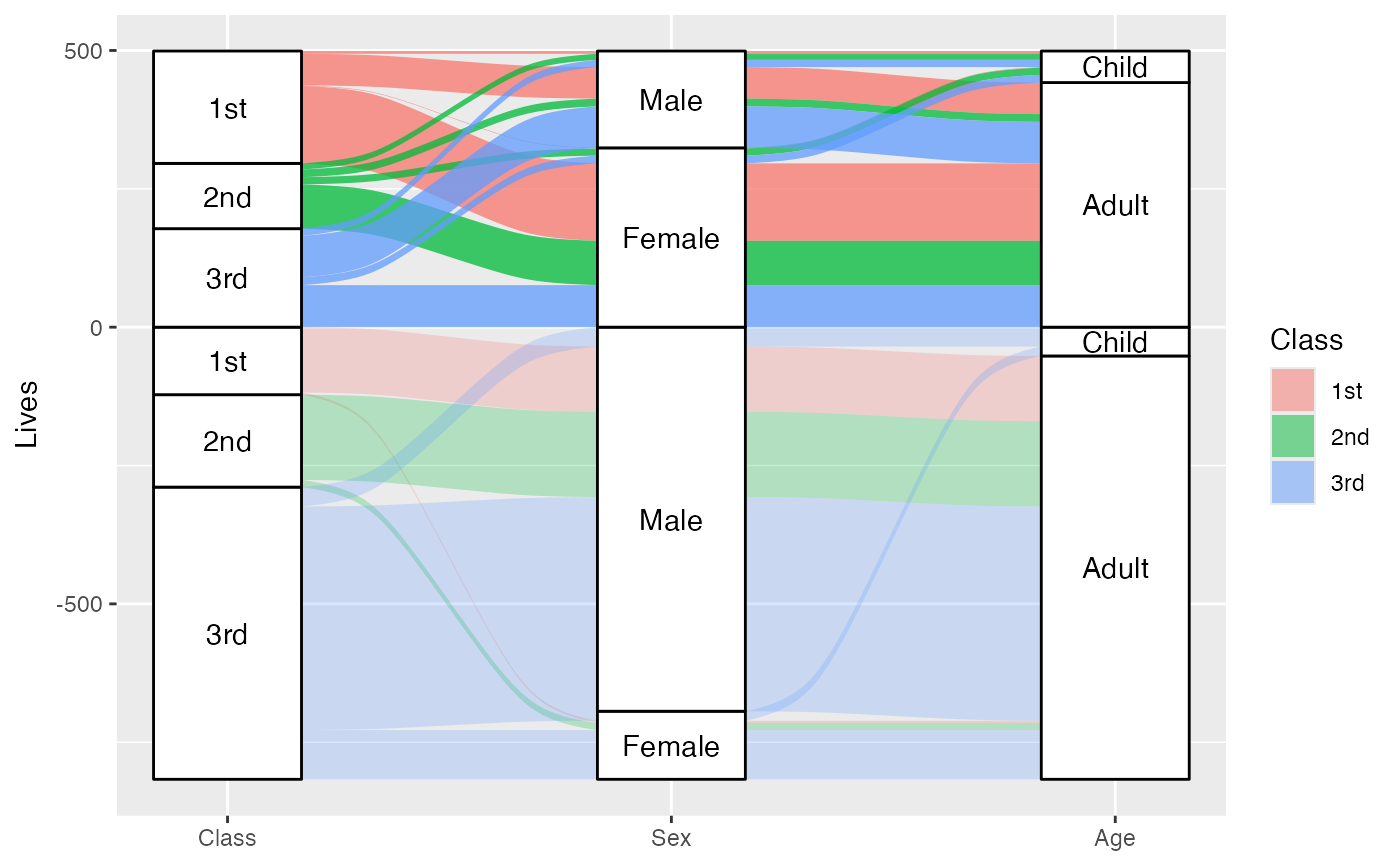# expanded to include missing values
bn2 <- merge(bn,
expand.grid(year = unique(bn$year), name = unique(bn$name)),
all = TRUE)
ggplot(data = bn2,
aes(x = year, alluvium = name, y = prop)) +
geom_alluvium(aes(fill = name, color = name == "Tammy"),
decreasing = TRUE, show.legend = FALSE) +
scale_color_manual(values = c("#00000000", "#000000"))
#> Warning: Removed 34 rows containing non-finite values (stat_alluvium()).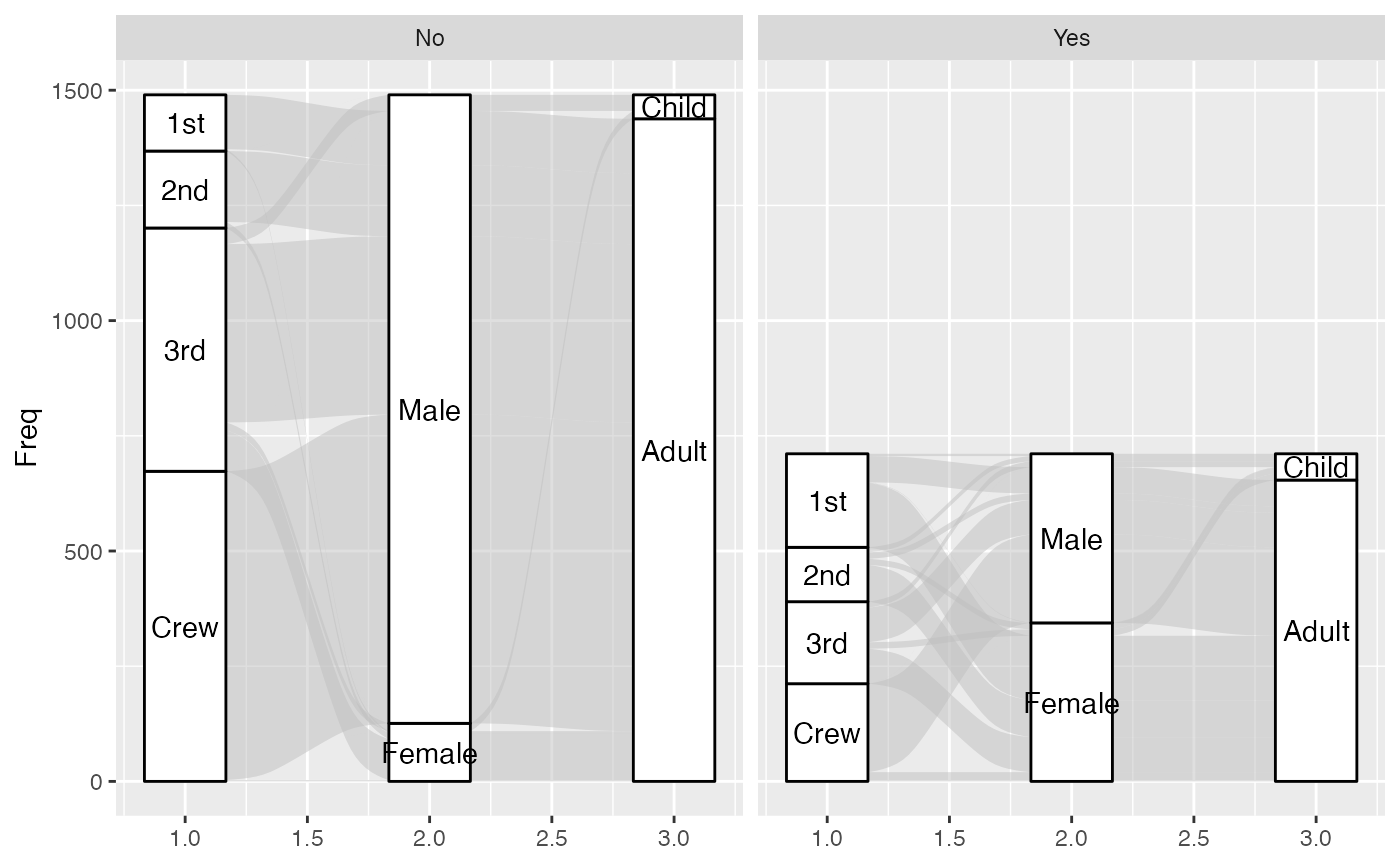# with missing values filled in with zeros
bn2$prop[is.na(bn2$prop)] <- 0
ggplot(data = bn2,
aes(x = year, alluvium = name, y = prop)) +
geom_alluvium(aes(fill = name, color = name == "Tammy"),
decreasing = TRUE, show.legend = FALSE) +
scale_color_manual(values = c("#00000000", "#000000"))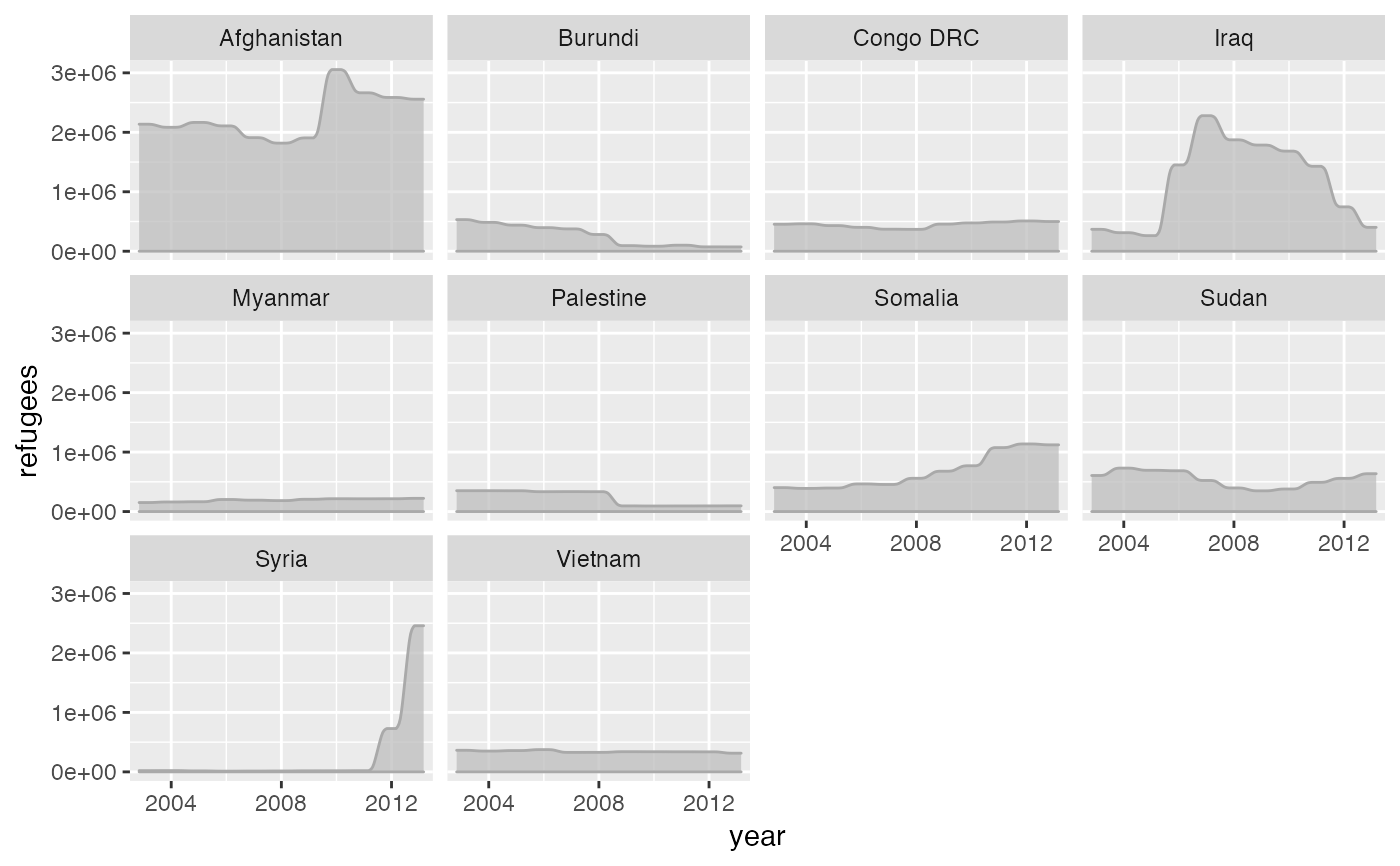# }

# use negative y values to encode deaths versus survivals
titanic <- as.data.frame(Titanic)
titanic <- transform(titanic, Lives = Freq * (-1) ^ (Survived == "No"))
ggplot(subset(titanic, Class != "Crew"),
aes(axis1 = Class, axis2 = Sex, axis3 = Age, y = Lives)) +
geom_alluvium(aes(alpha = Survived, fill = Class), absolute = FALSE) +
geom_stratum(absolute = FALSE) +
geom_text(stat = "stratum", aes(label = after_stat(stratum)),
absolute = FALSE) +
scale_x_discrete(limits = c("Class", "Sex", "Age"), expand = c(.1, .05)) +
scale_alpha_discrete(range = c(.25, .75), guide = "none")
#> Warning: Using alpha for a discrete variable is not advised.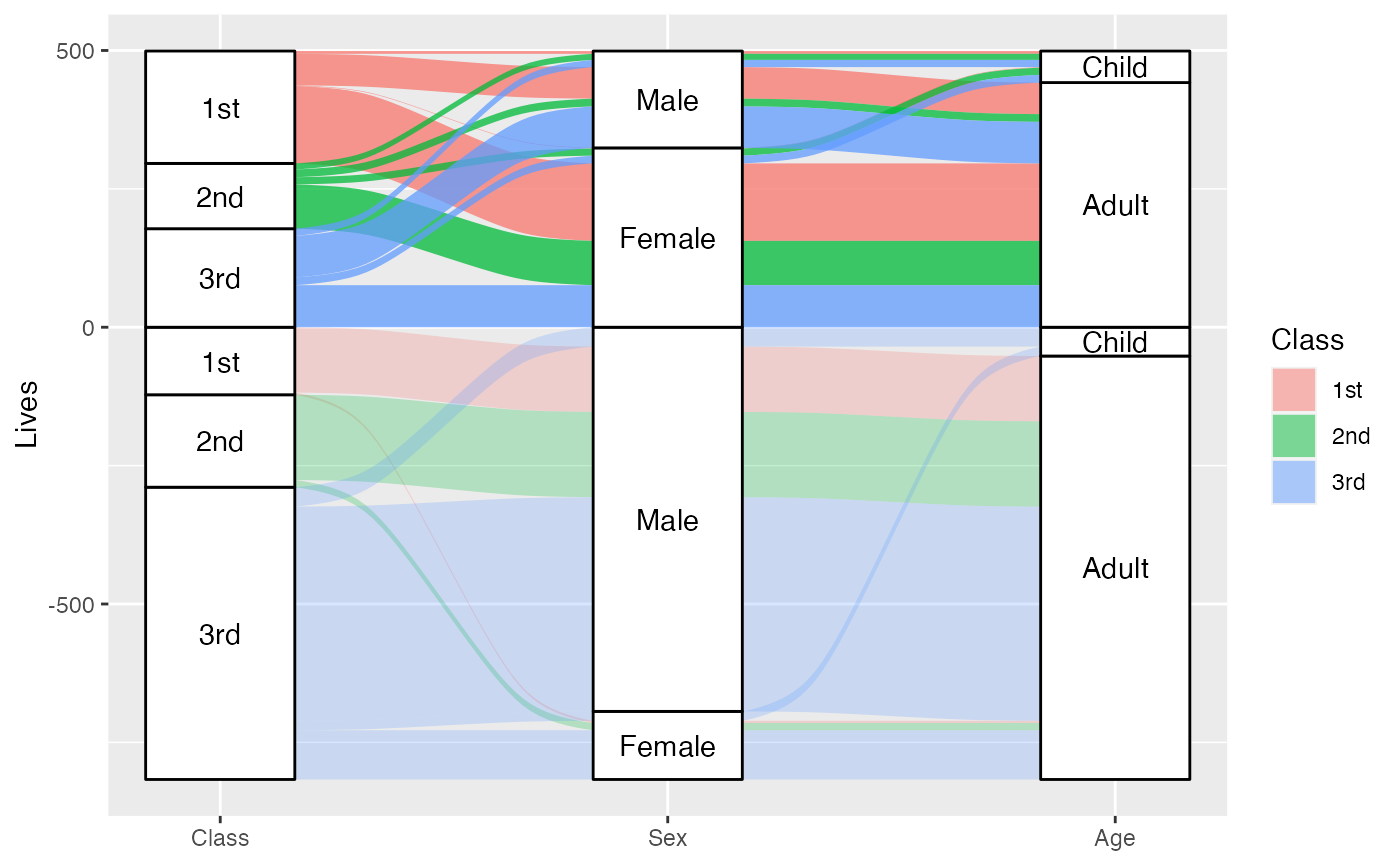# faceting with common alluvia
ggplot(titanic, aes(y = Freq, axis1 = Class, axis2 = Sex, axis3 = Age)) +
facet_wrap(~ Survived) +
geom_alluvium() +
geom_stratum() +
geom_text(stat = "stratum", aes(label = after_stat(stratum)))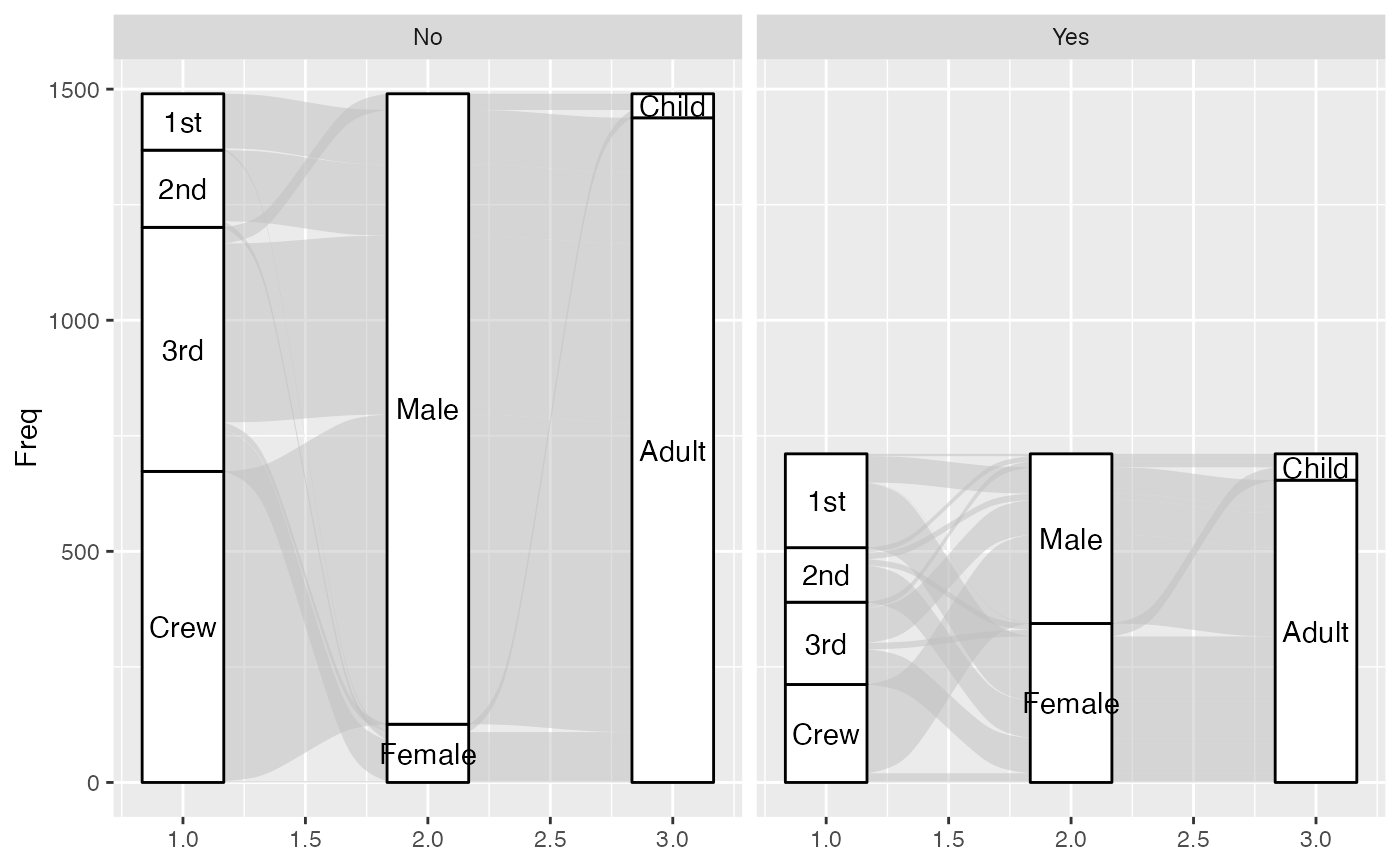ggplot(transform(alluvial::Refugees, id = 1),
aes(y = refugees, x = year, alluvium = id)) +
facet_wrap(~ country) +
geom_alluvium(alpha = .75, color = "darkgrey") +
scale_x_continuous(breaks = seq(2004, 2012, 4))